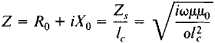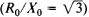# skin effect

Also found in: Dictionary, Thesaurus, Medical, Financial, Wikipedia.
Related to skin effect: Proximity effect

## Skin effect (electricity)

The tendency for an alternating current to concentrate near the outer part or “skin” of a conductor. For a steady unidirectional current through a homogeneous conductor, the current distribution is uniform over the cross section; that is, the current density is the same at all points in the cross section. With an alternating current, the current is displaced more and more to the surface as the frequency increases. The conductor's effective cross section is therefore reduced so the resistance and energy dissipation are increased compared with the values for a uniformly distributed current. The effective resistance of a wire rises significantly with frequency; for example, for a copper wire of 1-mm (0.04-in.) diameter, the resistance at a frequency of 1 MHz is almost four times the dc value. See Electrical resistance

A skin depth or penetration depth δ is frequently used in assessing the results of skin effect; it is the depth below the conductor surface at which the current density has decreased to 1/e (approximately 37%) of its value at the surface. This concept applies strictly only to plane solids, but can be extended to other shapes provided the radius of curvature of the conductor surface is appreciably greater than δ.

At a frequency of 60 Hz the penetration depth in copper is 8.5 mm (0.33 in.); at 10 GHz it is only 6.6 × 10-7 m. Wave-guide and resonant cavity internal surfaces for use at microwave frequencies are therefore frequently plated with a high-conductivity material, such as silver, to reduce the energy losses since nearly all the current is concentrated at the surface. Provided the plating material is thick compared to δ, the conductor is as good as a solid conductor of the coating material.

## Skin Effect

(also surface effect), the increased attenuation of electromagnetic waves as the waves penetrate into a conducting medium. As a result of this phenomenon, the alternating current over the cross section of a conductor or the alternating magnetic flux over the cross section of a magnetic circuit is not distributed uniformly but is located chiefly in the surface layer. The skin effect is due to the production of eddy currents when an electromagnetic wave propagates in a conducting medium, as a result of which part of the electromagnetic energy is converted into heat. This leads to a reduction in the intensity of the electric and magnetic fields and a reduction of the current density, that is, to an attenuation of the wave.

According to Maxwell’s equations, the higher the frequency v of the electromagnetic wave and the higher the magnetic permeability μ of the conductor, the stronger the rotational electric field caused by the alternating magnetic field. According to Ohm’s law and Joule’s law, the higher the conductivity σ of the conductor, the greater the current density and the power dissipated per unit volume. Thus, the larger ν, μ, and σ, the greater the attenuation, that is, the more marked the skin effect.

In the case of a plane sinusoidal wave propagating along the x-axis in a uniform, linear medium that is a good conductor (the displacement currents are negligible compared with the conduction currents), the amplitudes of the electric and magnetic field intensities are attenuated according to the exponential law:

E = E0e-αx and H = H0e-αx

whereis the coefficient of attenuation and μ0 is the magnetic constant. At a depth x = δ = 1/α, the amplitude of the wave is diminished by the factor e. This distance is called the depth of penetration or the skin depth. For example, at a frequency of 50 hertz in copper (σ = 580 kilosiemens/cm and μ = 1), δ = 9.4 mm; in steel (σ = 100 kilosiemens/cm and μ = 1,000), δ = 0.74 mm. When the frequency is increased to 0.5 megahertz, δ is reduced by a factor of 100. An electromagnetic wave does not penetrate at all into an ideal conductor (one with infinitely great conductivity) but is reflected completely from it. The shorter the distance that a wave travels compared with δ, the weaker the manifestation of the skin effect.

The concept of surface (skin) impedance Zs is introduced for conductors with a very pronounced skin effect when the radius of curvature of the conductor’s cross section is significantly greater than δ and the field in the conductor represents a plane wave. The skin impedance is defined as the ratio of the complex amplitude of the voltage drop per unit conductor length to the complex amplitude of the current in the cross section of the unit skin length. The complex impedance of the conductor per unit length iswhere R0 is the effective resistance of the conductor, which determines the power loss, X0 is the inductive reactance, which takes into account the inductance of the conductor resulting from the magnetic flux inside the conductor, lc is the perimeter of the skin’s cross section, and ω = 2πν; here, R0 = X0. When the skin effect is pronounced, the surface impedance is the same as the characteristic impedance of the conductor and, consequently, is equal to the ratio of the intensity of the electric field to the intensity of the magnetic field at the surface of the conductor.

In those cases where the length of the free path l for the current carriers becomes greater than the skin depth δ—for example, in very pure metals at low temperatures—the skin effect at relatively high frequencies acquires a number of characteristics and is called anomalous. Since the field over the length of the electron free path is nonuniform, the current at a given point depends not only on the value of the electric field for this point but also on the value of the region around the point having a dimension of the order of l. Consequently, when solving Maxwell’s equations it is necessary to use in place of Ohm’s law the Boltzmann kinetic equation in order to compute the current. With the anomalous skin effect, electrons become unequal from the standpoint of their contribution to the electric current. When l » δ, the principal contribution is made by the electrons that move in the skin parallel to the metal surface or at very small angles to the surface, thus spending a long time in the region of the strong field. The electromagnetic wave is attenuated in the surface layer as before, but the quantitative characteristics of the anomalous skin effect are somewhat different. The field in the skin is not attenuated exponentially.

In the infrared range of frequencies an electron is unable to travel the distance l during the period of a field alternation. In this case, the field can be considered uniform over the period for the path of the electron. This again leads to Ohm’s law, and the skin effect again becomes normal. Thus, at low and very high frequencies the skin effect is always normal. In the radio-frequency range the effect may be normal or anomalous, depending on the ratio of l to δ. All the above is valid as long as the frequency ω is lower than the plasma frequency: ω < ω0 ≈ (4πne2/m)½, where n is the concentration of free electrons, e is the charge, and m is the mass of an electron. (SeeMETAL OPTICS for higher frequencies.)

The skin effect is often undesirable. In conductors having a pronounced skin effect, alternating current flows mainly through the surface layer; the conductor’s cross section is not used to its full extent, and the resistance and power losses in the conductor for a given current are increased. In ferromagnetic laminae or strips for the magnetic circuits of transformers, electric machines, and other devices, the alternating magnetic flux in the case of a marked skin effect passes mainly through the surface layer. As a result, utilization of the cross section of the magnetic circuit becomes less efficient, the magnetizing current is increased, and the losses in the steel are higher. The objectionable influence of the skin effect is diminished by reducing the thickness of the laminae or strips and, when the frequencies are high enough, by using magnetic circuits made of magneto-dielectrics.

On the other hand, the skin effect has practical applications, for example, in the action of electromagnetic shields. In order to protect the surrounding space from the interference created by the field from a power transformer operating at a frequency of 50 hertz, a shield of comparatively thick ferromagnetic steel is used. In order to shield an inductance coil operating at high frequencies, the shields are made out of a thin layer of Al. The high-frequency surface hardening of steel objects is also based on the skin effect. (SeeINDUCTION HEATING PLANT.)

### REFERENCES

Netushil, A. V., and K. M. Polivanov. Osnovy elektrotekhniki, vol. 3, Moscow, 1956.
Polivanov, K. M. Teoreticheskie osnovy elektrotekhniki, part 3: Teoriia elektromagnitnogo polia. Moscow, 1975.
Neiman, L. R. Poverkhnostnyi effekt ν ferromagnilnykh telakh. Leningrad-Moscow, 1949.

I. B. NEGNEVITSKII

## skin effect

[′skin i‚fekt]
(electricity)
The tendency of alternating currents to flow near the surface of a conductor thus being restricted to a small part of the total sectional area and producing the effect of increasing the resistance. Also known as conductor skin effect; Kelvin skin effect.
(petroleum engineering)
The restriction to fluid flow through a reservoir adjacent to the borehole; calculated as a factor of reservoir pressure, product rate, formation volume and thickness, porosity, and other related parameters.

## skin effect

With alternating current (AC), electrons flow more at the outer surface of the wire rather than through the middle. The higher the frequency, the more the skin effect and the greater the resistance. Stranded wire produces less skin effect than solid, because there is more surface area. The skin effect enables copper-clad steel wire to be used. The steel adds cable strength, and the current flows mostly through the better-conducting copper. See also skin.
References in periodicals archive ?
Calculation for Skin Effect. We resolved the integration Maxwell-Faraday equation numerically and calculated the electric field generated by the current-generated magnetic field inside the metal.
Owing to skin effect, higher frequency results in less current near the center of the cable and the internal inductance decreases.
The standard approach usually employed to deal with time-domain skin effect problems involves a detour in the frequency-domain.
At the heart of the heating are two physical phenomena: the skin effect and the method of direct, discrete conversion of radiated electromagnetic energy into heat.
(5) ignores the skin effect. In other words, the induced current dl is assumed to be only the result of externally changing magnetic flux.
In particular, the laminated copper bus minimizes the impedance increase caused by skin effect that otherwise would occur at high frequencies.
The first part is skin effect which increases the electrical resistance of the conductor.
 Skin effect: A phenomenon observed when high-frequency current is flowing through a conductor, where the higher the frequency, the more current tends to concentrate near the surface of the conductor.
SKIN Don't be tempted to emulate the ghostly pale skin effect seen on the catwalk - you'll end up looking like too much like Morticia Adams.
However, this can cause higher losses due to the high-frequency currents in conductors and the load caused by the skin effect and higher eddy-currents.
Catholique de Louvain and his co-authors cover the fundamentals of electromagnetics as it applies to biological tissues and the skin effect, RF/microwave interactions including bioelectricity and tissue characterization, biological effects at the cell level as well as various systems and in conjunction with drugs, thermal therapy in terms of the heating principle and hyperthermia, EM-wave absorbers protecting the biological and medical environment and their applications, and RF/microwave delivery systems for therapeutic applications including ablation, perfusion chambers and measurement.

Site: Follow: Share:
Open / Close